Updating search results...

# 8 Results

View
Selected filters:
• WI.Math.Content.5.NF.B.3
RemixConditional Remix & Share Permitted
CC BY-NC-SA
Rating
0.0 stars

This lesson will be an introduction to the many areas where math is utilized in an iron foundry.

Subject:
Mathematics
Material Type:
Lesson
Author:
Lynn Aprill
Monica Wagner
10/10/2019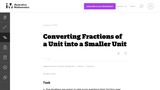Conditional Remix & Share Permitted
CC BY-NC-SA
Rating
0.0 stars

This task gives students word problems with a given a set of a specified size and a specified number of subsets. The questions ask the student to find out the size of each of the subsets.

Subject:
Mathematics
Measurement and Data
Numbers and Operations
Material Type:
Activity/Lab
Provider:
Illustrative Mathematics
Provider Set:
Illustrative Mathematics
Author:
Illustrative Mathematics
05/01/2012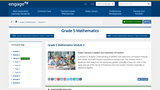Conditional Remix & Share Permitted
CC BY-NC-SA
Rating
0.0 stars

In Module 3, students' understanding of addition and subtraction of fractions extends from earlier work with fraction equivalence and decimals. This module marks a significant shift away from the elementary grades' centrality of base ten units to the study and use of the full set of fractional units from Grade 5 forward, especially as applied to algebra.

Subject:
Ratios and Proportions
Material Type:
Module
Provider:
New York State Education Department
Provider Set:
EngageNY
11/27/2012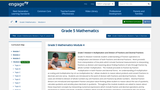Conditional Remix & Share Permitted
CC BY-NC-SA
Rating
0.0 stars

Grade 5s Module 4 extends student understanding of fraction operations to multiplication and division of both fractions and decimal fractions.  Work proceeds from interpretation of line plots which include fractional measurements to interpreting fractions as division and reasoning about finding fractions of sets through fraction by whole number multiplication.  The module proceeds to fraction by fraction multiplication in both fraction and decimal forms.  An understanding of multiplication as scaling and multiplication by n/n as multiplication by 1 allows students to reason about products and convert fractions to decimals and vice versa.  Students are introduced to the work of division with fractions and decimal fractions.  Division cases are limited to division of whole numbers by unit fractions and unit fractions by whole numbers.  Decimal fraction divisors are introduced and equivalent fraction and place value thinking allow student to reason about the size of quotients, calculate quotients and sensibly place decimals in quotients.  Throughout the module students are asked to reason about these important concepts by interpreting numerical expressions which include fraction and decimal operations and by persevering in solving real-world, multistep problems which include all fraction operations supported by the use of tape diagrams.

Subject:
Ratios and Proportions
Material Type:
Module
Provider:
New York State Education Department
Provider Set:
EngageNY
11/15/2013Conditional Remix & Share Permitted
CC BY-NC-SA
Rating
0.0 stars

The purpose of this task is to help students see the connection between aÖb and ab in a particular concrete example. The relationship between the division problem 3Ö8 and the fraction 3/8 is actually very subtle.

Subject:
Mathematics
Numbers and Operations
Material Type:
Activity/Lab
Provider:
Illustrative Mathematics
Provider Set:
Illustrative Mathematics
Author:
Illustrative Mathematics
07/15/2012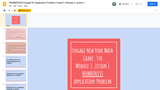Unrestricted Use
Public Domain
Rating
0.0 stars

*This numberless application problem is the same application problem used the engage lesson: Grade 5, Module 3, Lesson 1
*Numberless word problems are used to support students in solving word problems. They allow students to discover for themselves the structure of the problems they're solving. In doing so, they will be able to successfully find the operation or operations they need to use to determine the solution.
*Numberless word problems help students develop number sense, make sense of word problems, and encourage precise mathematical vocabulary.
*Based on the work of Brian Bushart.
*Created using ideas from WI Math Institute training. Google Slides presentation created as an adaption of WiseLearn resource by Sarah Martinsen and Kelly Shaefer.
*These slides could be inserted into the free Engage New York lesson google slides from embarc.online.

Subject:
Mathematics
Material Type:
Author:
Jessica DuBois
Megan Sherman
06/11/2019Conditional Remix & Share Permitted
CC BY-NC-SA
Rating
0.0 stars

The purpose of this task is for students to find the answer to a question in context that can be represented by fraction multiplication. This task is appropriate for either instruction or assessment depending on how it is used and where students are in their understanding of fraction multiplication.

Subject:
Mathematics
Numbers and Operations
Material Type:
Activity/Lab
Provider:
Illustrative Mathematics
Provider Set:
Illustrative Mathematics
Author:
Illustrative Mathematics
08/21/2012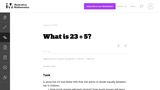Conditional Remix & Share Permitted
CC BY-NC-SA
Rating
0.0 stars

When a division problem involving whole numbers does not result in a whole number quotient, it is important for students to be able to decide whether the context requires the result to be reported as a whole number with remainder or a mixed number.

Subject:
Mathematics
Numbers and Operations
Material Type:
Activity/Lab
Provider:
Illustrative Mathematics
Provider Set:
Illustrative Mathematics
Author:
Illustrative Mathematics